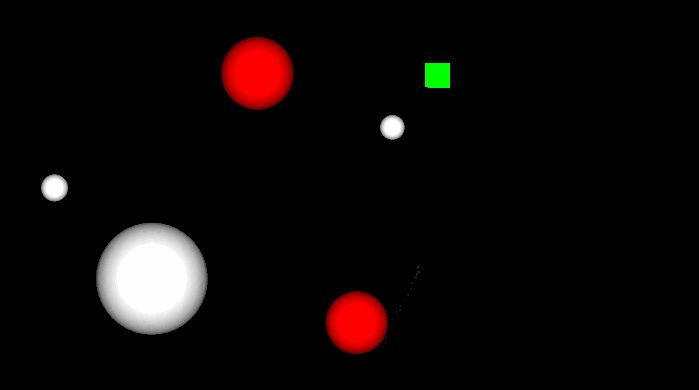# Making a Target Tracking Orthographic Camera in Unity

March 30, 2014

I’m working on a new prototype of a 2D local multiplayer space game. I wanted to make a camera system that allowed for larger levels, while keeping all players in view. Another requirement was that the camera zoomed in and out depending on player proximity to create a more dynamic view of the action.

I’m working in Unity, so the code below is in a `MonoBehaviour` class. It should be attached to a `GameObject` with the `Camera` component. This could be improved in many ways, but it served its purpose for my prototype. Below is a short gif that showing the camera tracking three targets (green and red), the code for the component, and short explanations of each method at the end of this post.``````using UnityEngine;

public class TrackTargets : MonoBehaviour {

[SerializeField]
Transform[] targets;

[SerializeField]

[SerializeField]
float minimumOrthographicSize = 8f;

[SerializeField]
float zoomSpeed = 20f;

Camera camera;

void Awake ()
{
camera = GetComponent<Camera>();
camera.orthographic = true;
}

void LateUpdate()
{
Rect boundingBox = CalculateTargetsBoundingBox();
transform.position = CalculateCameraPosition(boundingBox);
camera.orthographicSize = CalculateOrthographicSize(boundingBox);
}

/// <summary>
/// Calculates a bounding box that contains all the targets.
/// </summary>
/// <returns>A Rect containing all the targets.</returns>
Rect CalculateTargetsBoundingBox()
{
float minX = Mathf.Infinity;
float maxX = Mathf.NegativeInfinity;
float minY = Mathf.Infinity;
float maxY = Mathf.NegativeInfinity;

foreach (Transform target in targets) {
Vector3 position = target.position;

minX = Mathf.Min(minX, position.x);
minY = Mathf.Min(minY, position.y);
maxX = Mathf.Max(maxX, position.x);
maxY = Mathf.Max(maxY, position.y);
}

}

/// <summary>
/// Calculates a camera position given the a bounding box containing all the targets.
/// </summary>
/// <param name="boundingBox">A Rect bounding box containg all targets.</param>
/// <returns>A Vector3 in the center of the bounding box.</returns>
Vector3 CalculateCameraPosition(Rect boundingBox)
{
Vector2 boundingBoxCenter = boundingBox.center;

return new Vector3(boundingBoxCenter.x, boundingBoxCenter.y, camera.transform.position.z);
}

/// <summary>
/// Calculates a new orthographic size for the camera based on the target bounding box.
/// </summary>
/// <param name="boundingBox">A Rect bounding box containg all targets.</param>
/// <returns>A float for the orthographic size.</returns>
float CalculateOrthographicSize(Rect boundingBox)
{
float orthographicSize = camera.orthographicSize;
Vector3 topRight = new Vector3(boundingBox.x + boundingBox.width, boundingBox.y, 0f);
Vector3 topRightAsViewport = camera.WorldToViewportPoint(topRight);

if (topRightAsViewport.x >= topRightAsViewport.y)
orthographicSize = Mathf.Abs(boundingBox.width) / camera.aspect / 2f;
else
orthographicSize = Mathf.Abs(boundingBox.height) / 2f;

return Mathf.Clamp(Mathf.Lerp(camera.orthographicSize, orthographicSize, Time.deltaTime * zoomSpeed), minimumOrthographicSize, Mathf.Infinity);
}
}``````

`LateUpdate()` runs every frame, and it sets the values for the camera. It retrieves a bounding box that contains all targets, gets the camera position based on the bounding box, and sets the camera’s orthographic size based on the bounding box.

`CalculateTargetsBoundingBox()` does exactly as it’s name explains, and returns a `Rect` that represents a box that fits all the targets. This is calculated by iterating through the targets array and retrieving the minimum and maximum x and y positions from those `GameObjects`. It also adds a padding to the bounding box so that the camera view will adjust before the targets hit the edge.

`CalculateCameraPosition()` is a simple method that simply returns the center of the target bounding box to use as the new camera location.

`CalculateOrthographicSize()` figures out the new orthographic size of the camera, based the bounding box the contains all the targets. Since the viewport of the camera and the bounding box are both centered and aligned rectangles, we can simply use one point of the bounding box to get the new orthographic size. Using `camera.WorldToViewportPoint(topRight)` we get the top right point of the bounding box in Viewport form which basically gives us a ratio to work with. We then use this number to calculate the new orthographic size based on how the targets are moving and the shape of the target bounding box. Once we have the new orthographic size, we use a `Lerp` to more smoothly zoom the camera, while using `Clamp` to ensure we maintain a minimum orthographic size.

I’ve tested this with one to four simultaneous targets, and it seems to work quite well. With one target it simple behaves like a camera that’s locked to the target and follows it around the world.

Hopefully this simple explanation is enough to get your started with the camera. If you have any further questions please feel free to message me on Twitter @RyanNielson or comment on this post’s page.